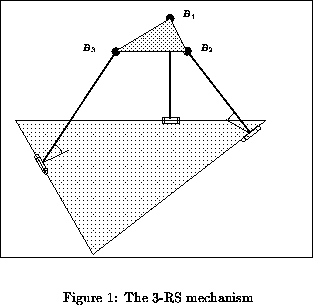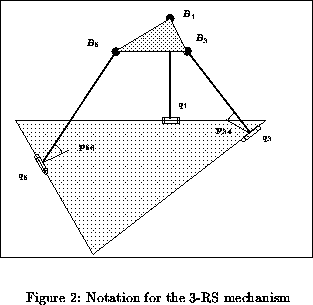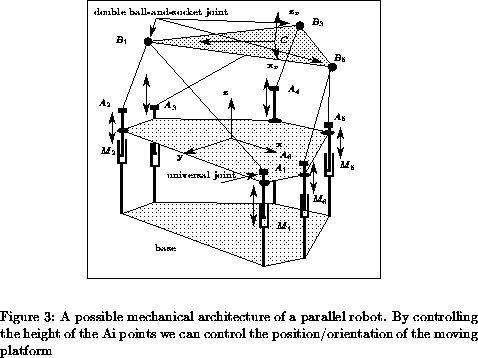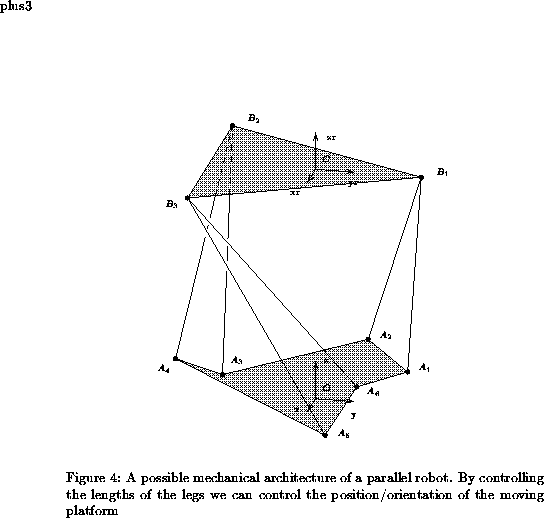•HEPHAISTOS Home page
•INRIA Home page

# Purpose

FK-3-(RS) is a package which enable to compute the solutions of the forward kinematics of 3-(RS) mechanism.

For many parallel manipulators the direct kinematics can be reduced to solving the forward kinematics of a 3-(RS): for example the 6-3 robots, the Stewart platforms, the HEXA robots or the INRIA prototype have a forward kinematics which is completely equivalent to solve a 3-(RS) mechanism. As example programs using this package for solving the 6-3 robots and the INRIA prototype forward kinematics are included.

# Availability

Free source codes are available under Free Software Society conventions through anonymous ftp (download here).

This software is provided "as is" without warranty of any kind. In no event shall INRIA be liable for any loss of profits, loss of business, loss of use or data, interruption of business, or for indirect, special, incidental, or consequential damages of any kind, arising from any error in this software. No commercial use of this software can be made without the previous agreement of INRIA. The use of this software for any publication should acknowledged the contribution of INRIA.

# Implementation features

Window system: none
Type: sources
Language: C
Number of lines: 9086
Size of binary: 2.66 Mo
Output: results, files, graphic output in xjpdraw format

# Introduction

FK-3-(RS) is a package which enable to compute the solutions of the forward kinematics of 3-(RS) mechanism (figure 1).The 3-(RS) mechanism is constituted of a base and a moving platform, which is a triangle. Each vertex of this triangle is connected to the ground by a fixed length link which is attached to the platform by a ball-and-socket joint. The other extremity of the link is connected to the ground through a revolute joint.

For many parallel manipulators the direct kinematics can be reduced to solving the forward kinematics of a 3-(RS): for example the 6-3 robots, the Stewart platforms, the HEXA robots or the INRIA prototype have a forward kinematics which is completely equivalent to solve a 3-(RS) mechanism.

There may be up to 16 different postures for the moving platform of the 3-(RS) mechanism . The package enables to compute a 16th order polynomial, which in turn enable to compute all the possible postures.

A 3-RS mechanism is defined in a structure:

```
/* position of the base revolute joint center */
double q1;
double q3;
double q5;
/* a possible position of the platform joints (optional) */
double p1,p3,p5;
/* the 3 angles associated to the previous positions (optional)*/
double p12,p34,p56;
double r12,r34,r56;
/* distances between B1B3, B1B5, B3B5 */
double l13,l15,l35;
/* unit vector of the revolute joint axis */
double g12,g34,g56;
/* vectors which constitutes with gij an orthonormal basis and
such that if p_ij=0 then p_iq_i= r_ij u_ij and u_ij=0 */
double u12,v12,u34,v34,u56,v56;
with the notation of figure 2q1,q3,a5 are the coordinates of the revolute joint centers and g12, g34, g56 are unit vector defining the axis of the revolute joints. Together with uij, vij they define three orthonormal basis. r12, r34, r56 are the link lengths. The angles between the links and the base are denoted p12, p34, p56. The triangles is defined by the distances l13 between B1, B3, l15 between B1, B5 and l35 between B3, B5.

As soon as such a structure is filed you can call the procedure:

```
where rssr is the mechanism structure, coeff is the coefficient of the polynomial by increasing power (the unknown of this polynomial is the tangent of p12/2). sol_pijare the possible values of the angles p12, p34, p56. posij is the possible locations of the point B1, B3, B5 and eps is an accuracy threshold which enable to dismiss the solutions such that the difference between the distances between B1, B3, B5 and their real value are greater than eps. This threshold should be a small number to avoid spurious solutions.

A easy way to define a 3-(RS) structure is to use the program:

```
Assume that you have a robot that have 6 joints Ai on the base and 3 joints Bi on the platform. Suppose that A1, A2 are connected by fixed length links to B1: then B1 lie on a circle whose center is located on the line A1, A2 and whose radius can be computed from the geometry of the triangle A1, A2, B1. If the same assumption hold for A3, A4, B3 and A5, A6, B5 then we get a 3-(RS) mechanism. Assume now that you known the coordinates in the absolute frame of the Ai, Bi. You fill face1 with the coordinates of A1, A2, B1, faces with the coordinates of A3, A4, B3 and faces with the coordinates of A5, A6, B5. Then the program will compute for you the 3-(RS) structure.

An example is given in the program verif.c which will compute for you the solution of a given 3-(RS) mechanism, together with some informations on the resolution: number of real solutions of the polynomial, number of identical solutions, number of solutions rejected due to errors on the distances between the point Bi.

# Application examples

## proto_inria

This program enables to solve the forward kinematics problem for the architecture described in figure 3), i.e. being given the height of the Ai points of the legs compute the position and orientation of the moving platform.Here the leg lengths are constant. For the forward kinematics the position of the Ai points are fixed and consequently the mechanism is equivalent to a 3-(RS) mechanism.

Such robot is defined in a mechanism file which contain the following data (see figure 3):

```
xa1 ya1 za1
xa2 ya2 za2
........
xb1 yb1 zb1
xb2 yb2 zb2
.........
l1 l2 l3 l4 l5 l6```
Where xai,yai,zai are the coordinates of points Ai (zai should be equal to 0), xbi, ybi, zbi are the coordinates of points Bi (zbi should be 0 and B1=B2, B3=B4, B5=B6) and li are the leg lengths.

Note that such file is compatible with the visualization program visu_robot also available via ftp.

### Results

You run the program with the name of a robot file as an argument.

Then you enter in the following menu:

```
number of assembly modes (input:cartesian)      :(0)?
angles p12,p34,p56 (input:cartesian)            :(1)?
polynomial coefficients (input:cartesian)       :(2)?
jpdraw file for polynomial (input:cartesian)    :(3)?
possible coordinates of Bi (input:cartesian)    :(4)?
debug of a solution (input:cartesian)           :(5)?
number of assembly modes (input:articular)      :(6)?```
Using 0 the program will ask you a position/orientation, then it will compute the corresponding height of the Ai and then solve the forward kinematics with these data (you should therefore find your initial position among the solutions). If you use 5 the procedure is the same except that the program displays the number of real solution to the polynomial.

Using 1 the program will ask you a position/orientation, then it will compute the corresponding height of the Ai and then solve the forward kinematics and display the possible angles (p12, p34, p56).

Using 2 the program will ask you a position/orientation, then will display the coefficients of the polynomial which has to be solved for the forward kinematics.

Using 3 you will have a graphical plot of the polynomial in the xjpdraw graphical format (xjpdraw is available via ftp). The program will ask you the range for the unknown of the univariate polynomial (which is here given in degree), then a position/orientation. The name of the jpdraw file is poly.jpd. To see the polynomial use the command:

```
xjpdraw -X 7 -Y 6 -F poly.jpd```
where the arguments of X, Y are the width and height of the box which will enclose the drawing.

Using 4 the program will ask you a position/orientation, then it will compute the corresponding height of the Ai and then solve the forward kinematics and display the possible locations of (B1, B2, B3).

Using 6 the program will ask you the 6 heights of the Ai and then solve the forward kinematics with these data.

## 6-3

This program enables to solve the forward kinematics problem for the architecture described in figure 4), i.e. being given the lengths of the legs compute the position and orientation of the moving platform.For the forward kinematics the mechanism is equivalent to a 3-(RS) mechanism.

Such robot is defined in a mechanism file which contain the following data:

```
xa1 ya1 za1
xa2 ya2 za2
........
xb1 yb1 zb1
xb2 yb2 zb2
.........```
Where xai,yai,zai are the coordinates of points Ai, xbi, ybi, zbi are the coordinates of points Bi (zbi should be 0 and B1=B2, B3=B4, B5=B6).

Note that such file is compatible with the visualization program visu_robot also available via ftp.

### Results

You run the program with the name of a robot file as an argument.

Then you enter in the following menu:

```
solutions (input:cartesian)      :(0)?
solutions (input:articular)      :(1)?```
Using 0 the program will ask you a position/orientation, then it will compute the corresponding lengths of the leg and then solve the forward kinematics with these data (you should therefore find your initial position among the solutions).

Using 1 the program will ask you a 6 leg lengths and then solve the forward kinematics.

DATE

## References

1
Merlet J-P. Direct kinematics and assembly modes of parallel manipulators. International Journal of Robotics Research, 11(2):150-162, 1992.

•HEPHAISTOS Home page
•INRIA Home page

Jean-Pierre.Merlet@sophia.inria.fr
Tue Sep 12 20:16:03 MET DST 1995